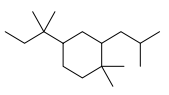Problem: Please answer the following questions for the compound given below.a) Provide a nameb) How many methine groups are there?c) Give the molecular formula for this compoundd) For the number of Cs that you gave in the moleular formula for this problem, what is the maximum possible number of Hs?e) How many secondary Hydrogens are there?f) How many quaternary Cs are there?

FREE Expert Solution
79% (271 ratings)
Problem Details

a) Provide a name

b) How many methine groups are there?

c) Give the molecular formula for this compound

d) For the number of Cs that you gave in the moleular formula for this problem, what is the maximum possible number of Hs?

e) How many secondary Hydrogens are there?

f) How many quaternary Cs are there?